# Building Java Programs Chapter 3 Parameters and Objects

• Slides: 53Redundant recipes • Recipe for baking 20 cookies: – Mix the following ingredients in a bowl: • 4 cups flour • 1 cup butter • 1 cup sugar • 2 eggs • 40 pounds chocolate chips. . . – Place on sheet and Bake for about 10 minutes. • Recipe for baking 40 cookies: – Mix the following ingredients in a bowl: • 8 cups flour • 2 cups butter • 2 cups sugar • 4 eggs • 80 pounds chocolate chips. . . – Place on sheet and Bake for about 10 minutes. 2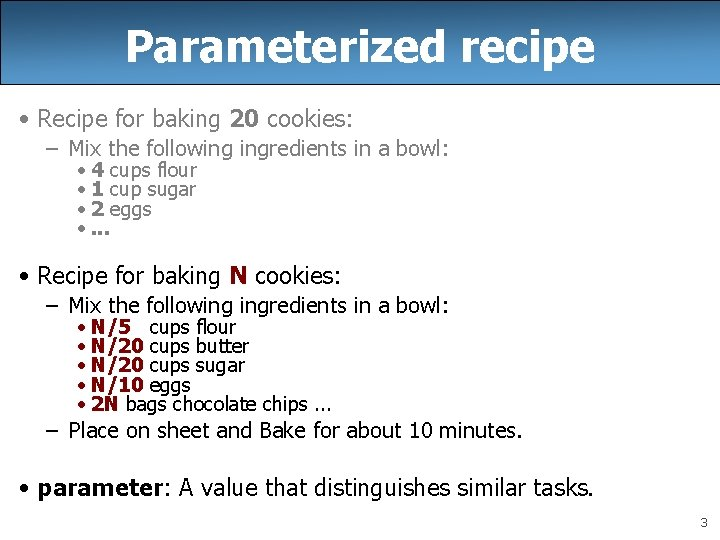Parameterized recipe • Recipe for baking 20 cookies: – Mix the following ingredients in a bowl: • 4 cups flour • 1 cup sugar • 2 eggs • . . . • Recipe for baking N cookies: – Mix the following ingredients in a bowl: • N/5 cups flour • N/20 cups butter • N/20 cups sugar • N/10 eggs • 2 N bags chocolate chips. . . – Place on sheet and Bake for about 10 minutes. • parameter: A value that distinguishes similar tasks. 3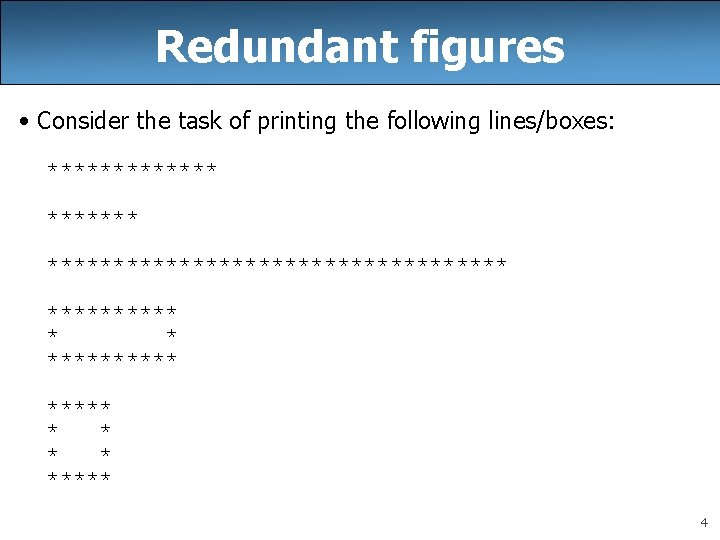Redundant figures • Consider the task of printing the following lines/boxes: ********************* ***** * * ***** 4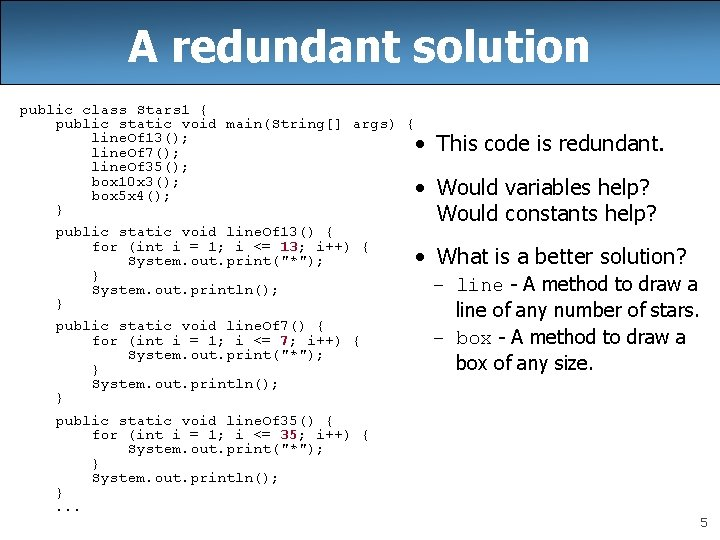A redundant solution public class Stars 1 { public static void main(String[] args) { line. Of 13(); • line. Of 7(); line. Of 35(); box 10 x 3(); • box 5 x 4(); } public static void line. Of 13() { for (int i = 1; i <= 13; i++) { • System. out. print("*"); } System. out. println(); } public static void line. Of 7() { for (int i = 1; i <= 7; i++) { System. out. print("*"); } System. out. println(); } public static void line. Of 35() { for (int i = 1; i <= 35; i++) { System. out. print("*"); } System. out. println(); }. . . This code is redundant. Would variables help? Would constants help? What is a better solution? – line - A method to draw a line of any number of stars. – box - A method to draw a box of any size. 5Parameterization • parameter: A value passed to a method by its caller. – Instead of line. Of 7, line. Of 13, write line to draw any length. • When declaring the method, we will state that it requires a parameter for the number of stars. • When calling the method, we will specify how many stars to draw. main 7 13 line ************* 6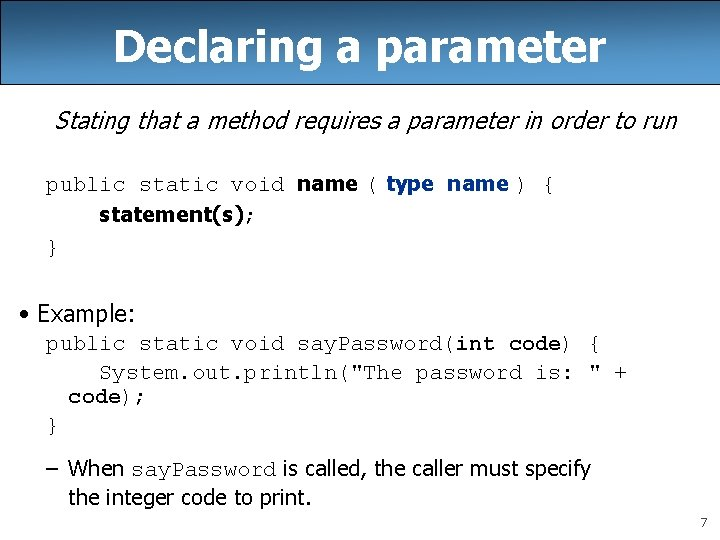Declaring a parameter Stating that a method requires a parameter in order to run public static void name ( type name ) { statement(s); } • Example: public static void say. Password(int code) { System. out. println("The password is: " + code); } – When say. Password is called, the caller must specify the integer code to print. 7Passing a parameter Calling a method and specifying values for its parameters name (expression); • Example: public static void main(String[] args) { say. Password(42); say. Password(12345); } Output: The password is 42 The password is 12345 8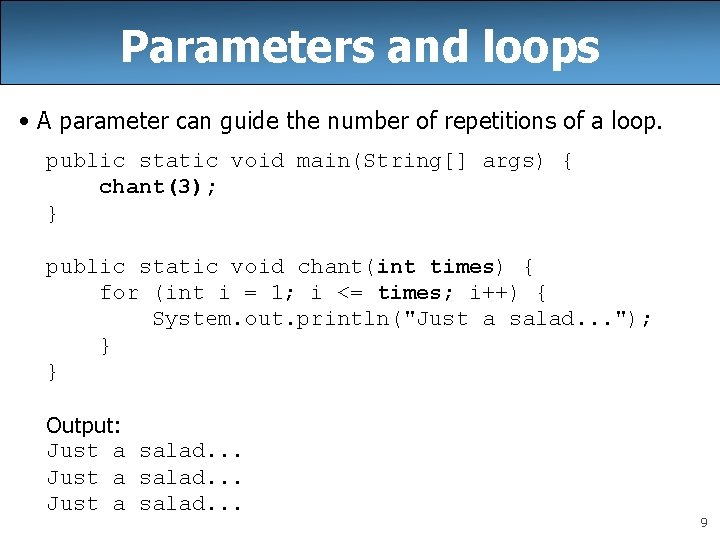Parameters and loops • A parameter can guide the number of repetitions of a loop. public static void main(String[] args) { chant(3); } public static void chant(int times) { for (int i = 1; i <= times; i++) { System. out. println("Just a salad. . . "); } } Output: Just a salad. . . 9How parameters are passed • When the method is called: – The value is stored into the parameter variable. – The method's code executes using that value. public static void main(String[] args) { chant(3); chant(7); } 3 7 public static void chant(int times) { for (int i = 1; i <= times; i++) { System. out. println("Just a salad. . . "); } } 10Common errors • If a method accepts a parameter, it is illegal to call it without passing any value for that parameter. chant(); // ERROR: parameter value required • The value passed to a method must be of the correct type. chant(3. 7); // ERROR: must be of type int • Exercise: Change the Stars program to use a parameterized method for drawing lines of stars. 11Stars solution // Prints several lines of stars. // Uses a parameterized method to remove redundancy. public class Stars 2 { public static void main(String[] args) { line(13); line(7); line(35); } // Prints the given number of stars plus a line break. public static void line(int count) { for (int i = 1; i <= count; i++) { System. out. print("*"); } System. out. println(); } } 12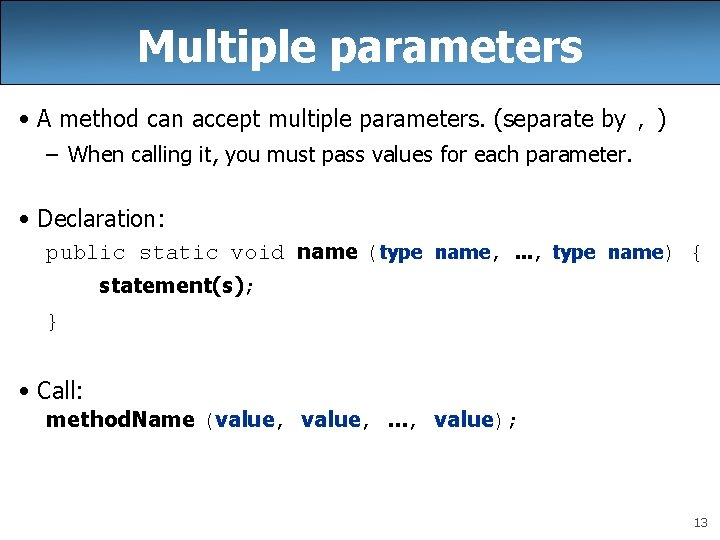Multiple parameters • A method can accept multiple parameters. (separate by , ) – When calling it, you must pass values for each parameter. • Declaration: public static void name (type name, . . . , type name) { statement(s); } • Call: method. Name (value, . . . , value); 13Multiple params example public static void main(String[] args) { print. Number(4, 9); print. Number(17, 6); print. Number(8, 0); print. Number(0, 8); } public static void print. Number(int number, int count) { for (int i = 1; i <= count; i++) { System. out. print(number); } System. out. println(); } Output: 44444 171717 0000 • Modify the Stars program to draw boxes with parameters. 14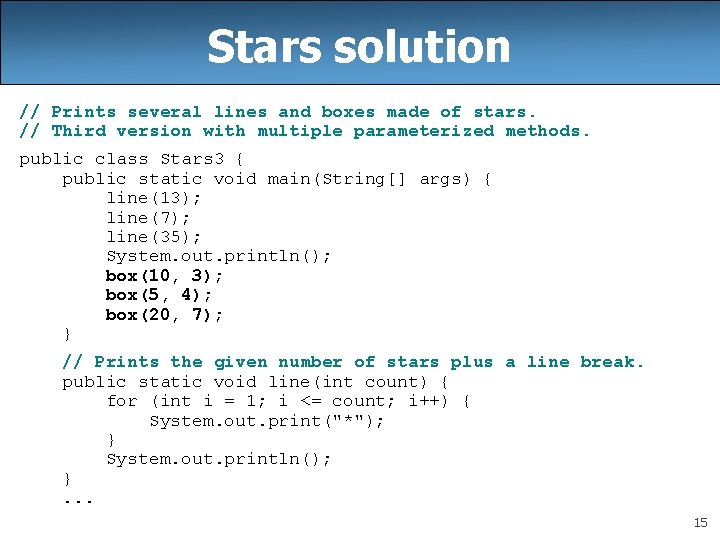Stars solution // Prints several lines and boxes made of stars. // Third version with multiple parameterized methods. public class Stars 3 { public static void main(String[] args) { line(13); line(7); line(35); System. out. println(); box(10, 3); box(5, 4); box(20, 7); } // Prints the given number of stars plus a line break. public static void line(int count) { for (int i = 1; i <= count; i++) { System. out. print("*"); } System. out. println(); }. . . 15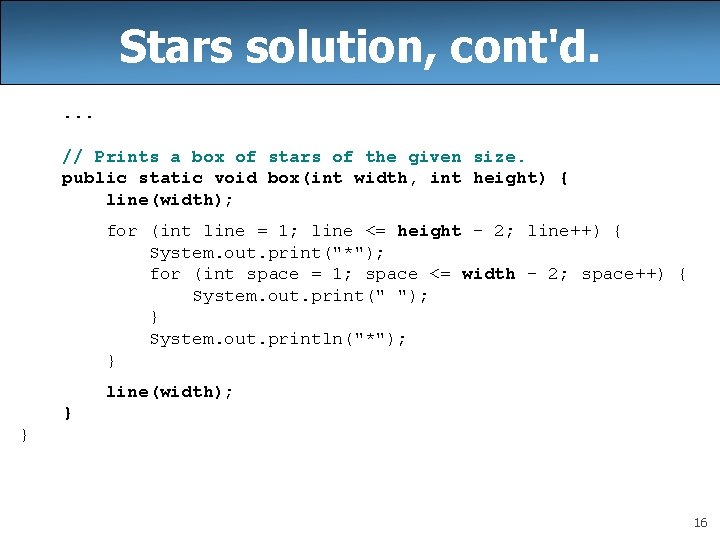Stars solution, cont'd. . // Prints a box of stars of the given size. public static void box(int width, int height) { line(width); for (int line = 1; line <= height - 2; line++) { System. out. print("*"); for (int space = 1; space <= width - 2; space++) { System. out. print(" "); } System. out. println("*"); } line(width); } } 16Value semantics • value semantics: When primitive variables (int, double) are passed as parameters, their values are copied. – Modifying the parameter will not affect the variable passed in. public static void strange(int x) { x = x + 1; System. out. println("1. x = " + x); } public static void main(String[] args) { int x = 23; strange(x); System. out. println("2. x = " + x); . . . } Output: 1. x = 24 2. x = 23 17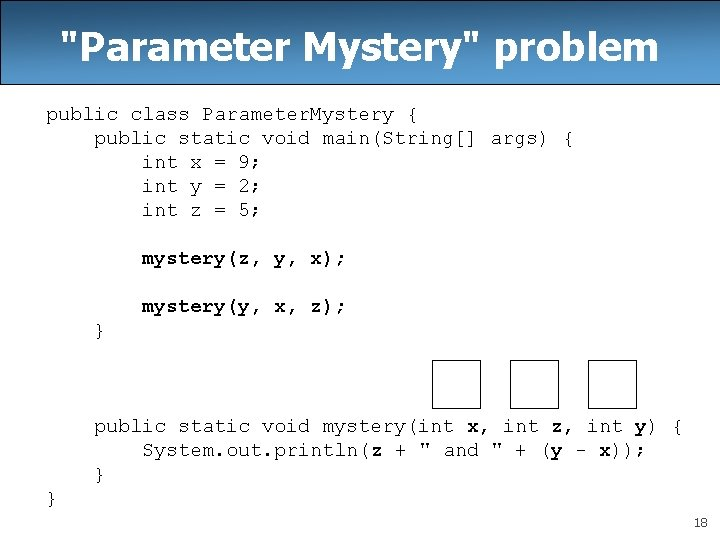"Parameter Mystery" problem public class Parameter. Mystery { public static void main(String[] args) { int x = 9; int y = 2; int z = 5; mystery(z, y, x); mystery(y, x, z); } public static void mystery(int x, int z, int y) { System. out. println(z + " and " + (y - x)); } } 18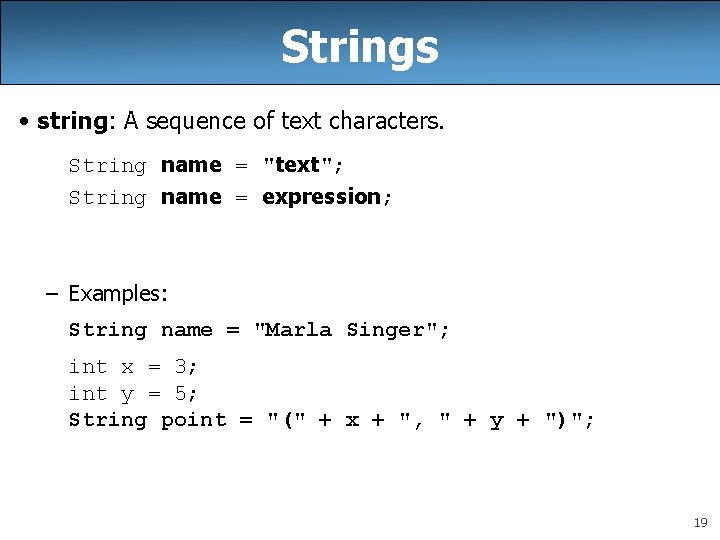Strings • string: A sequence of text characters. String name = "text"; String name = expression; – Examples: String name = "Marla Singer"; int x = 3; int y = 5; String point = "(" + x + ", " + y + ")"; 19Strings as parameters public class String. Parameters { public static void main(String[] args) { say. Hello("Marty"); String teacher = "Bictolia"; say. Hello(teacher); } public static void say. Hello(String name) { System. out. println("Welcome, " + name); } } Output: Welcome, Marty Welcome, Bictolia • Modify the Stars program to use string parameters. Use a method named repeat that prints a string many times. 20Stars solution // Prints several lines and boxes made of stars. // Fourth version with String parameters. public class Stars 4 { public static void main(String[] args) { line(13); line(7); line(35); System. out. println(); box(10, 3); box(5, 4); box(20, 7); } // Prints the given number of stars plus a line break. public static void line(int count) { repeat("*", count); System. out. println(); }. . . 21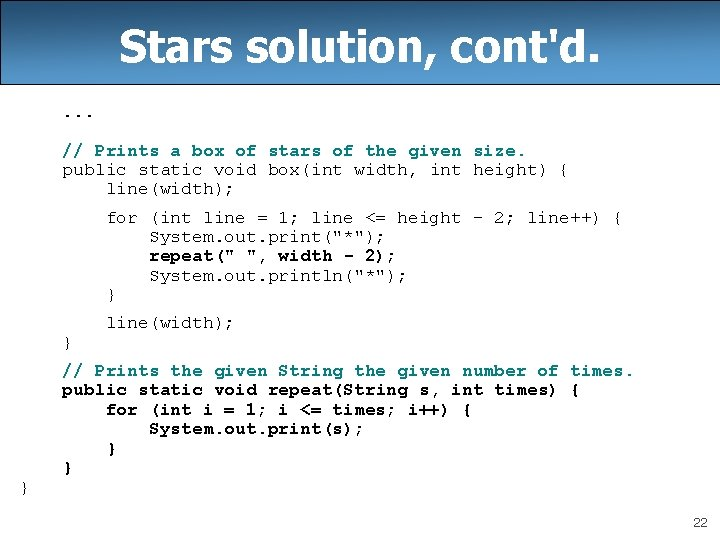Stars solution, cont'd. . // Prints a box of stars of the given size. public static void box(int width, int height) { line(width); for (int line = 1; line <= height - 2; line++) { System. out. print("*"); repeat(" ", width - 2); System. out. println("*"); } } } line(width); // Prints the given String the given number of times. public static void repeat(String s, int times) { for (int i = 1; i <= times; i++) { System. out. print(s); } } 22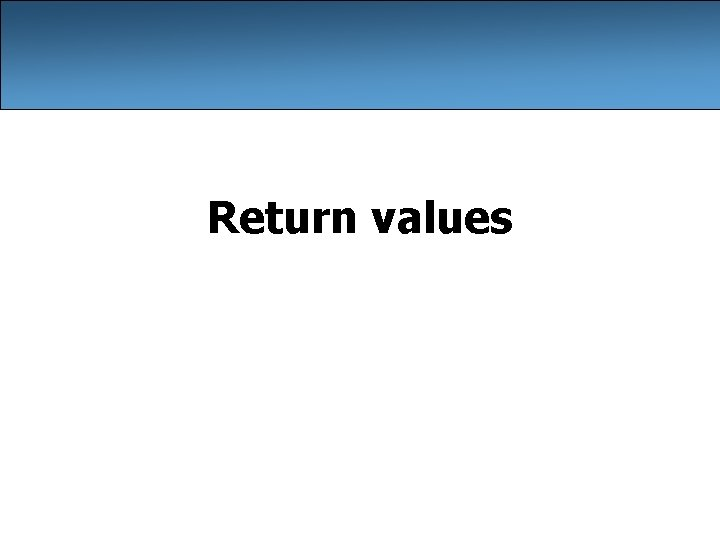Return values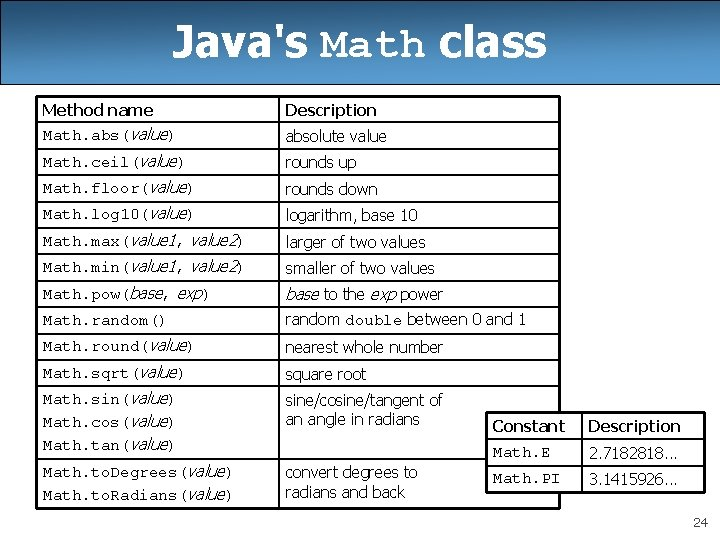Java's Math class Method name Description Math. abs(value) absolute value Math. ceil(value) rounds up Math. floor(value) rounds down Math. log 10(value) logarithm, base 10 Math. max(value 1, value 2) larger of two values Math. min(value 1, value 2) smaller of two values Math. pow(base, exp) base to the exp power Math. random() random double between 0 and 1 Math. round(value) nearest whole number Math. sqrt(value) square root Math. sin(value) Math. cos(value) Math. tan(value) sine/cosine/tangent of an angle in radians Math. to. Degrees(value) Math. to. Radians(value) convert degrees to radians and back Constant Description Math. E 2. 7182818. . . Math. PI 3. 1415926. . . 24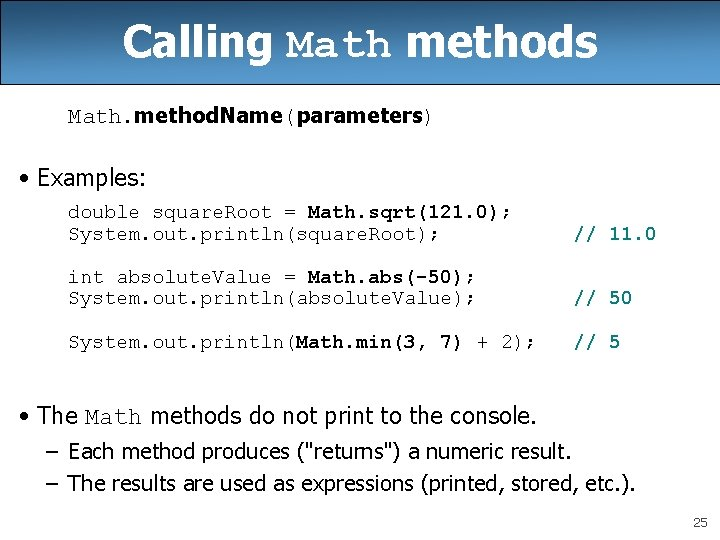Calling Math methods Math. method. Name(parameters) • Examples: double square. Root = Math. sqrt(121. 0); System. out. println(square. Root); // 11. 0 int absolute. Value = Math. abs(-50); System. out. println(absolute. Value); // 50 System. out. println(Math. min(3, 7) + 2); // 5 • The Math methods do not print to the console. – Each method produces ("returns") a numeric result. – The results are used as expressions (printed, stored, etc. ). 25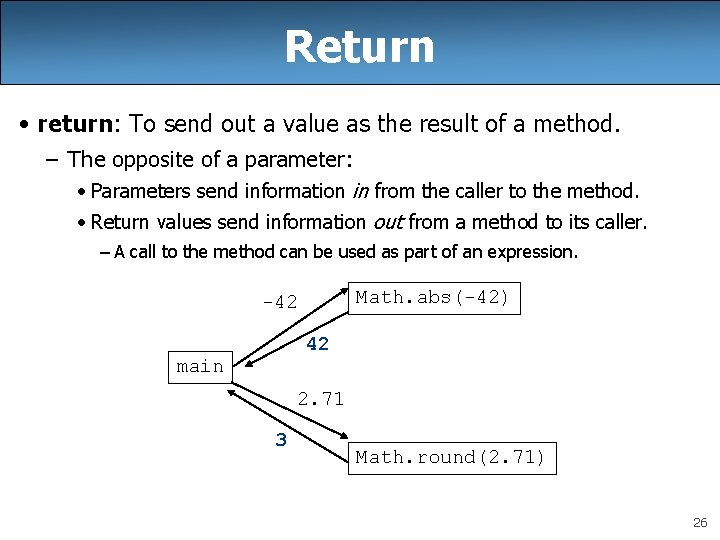Return • return: To send out a value as the result of a method. – The opposite of a parameter: • Parameters send information in from the caller to the method. • Return values send information out from a method to its caller. – A call to the method can be used as part of an expression. Math. abs(-42) -42 42 main 2. 71 3 Math. round(2. 71) 26Math questions • Evaluate the following expressions: – – – – Math. abs(-1. 23) Math. pow(3, 2) Math. pow(10, -2) Math. sqrt(121. 0) - Math. sqrt(256. 0) Math. round(Math. PI) + Math. round(Math. E) Math. ceil(6. 022) + Math. floor(15. 9994) Math. abs(Math. min(-3, -5)) • Math. max and Math. min can be used to bound numbers. Consider an int variable named age. – What statement would replace negative ages with 0? – What statement would cap the maximum age to 40? 27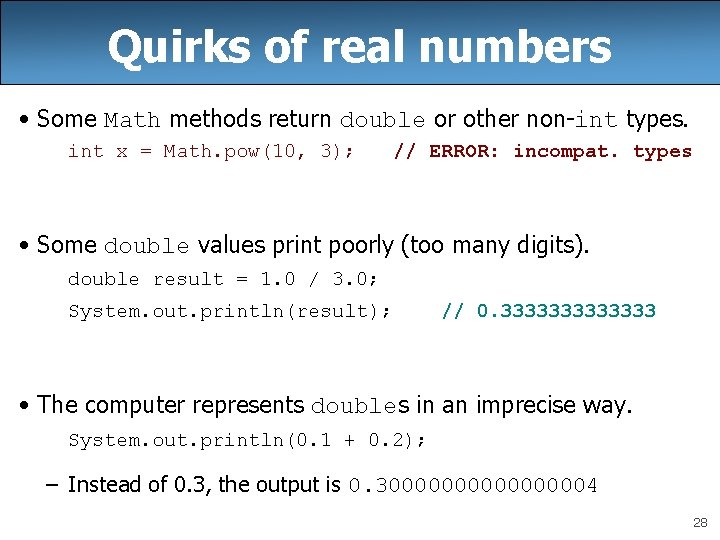Quirks of real numbers • Some Math methods return double or other non-int types. int x = Math. pow(10, 3); // ERROR: incompat. types • Some double values print poorly (too many digits). double result = 1. 0 / 3. 0; System. out. println(result); // 0. 3333333 • The computer represents doubles in an imprecise way. System. out. println(0. 1 + 0. 2); – Instead of 0. 3, the output is 0. 3000000004 28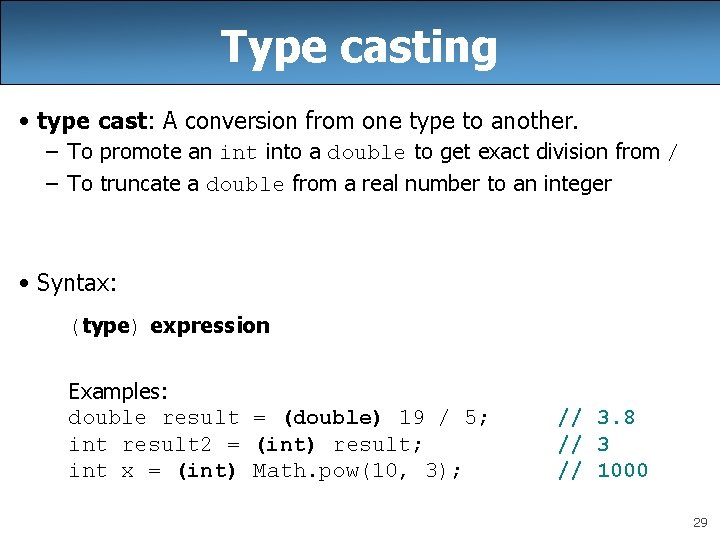Type casting • type cast: A conversion from one type to another. – To promote an into a double to get exact division from / – To truncate a double from a real number to an integer • Syntax: (type) expression Examples: double result = (double) 19 / 5; int result 2 = (int) result; int x = (int) Math. pow(10, 3); // 3. 8 // 3 // 1000 29More about type casting • Type casting has high precedence and only casts the item immediately next to it. – double x = (double) 1 + 1 / 2; – double y = 1 + (double) 1 / 2; // 1. 5 • You can use parentheses to force evaluation order. – double average = (double) (a + b + c) / 3; • A conversion to double can be achieved in other ways. – double average = 1. 0 * (a + b + c) / 3; 30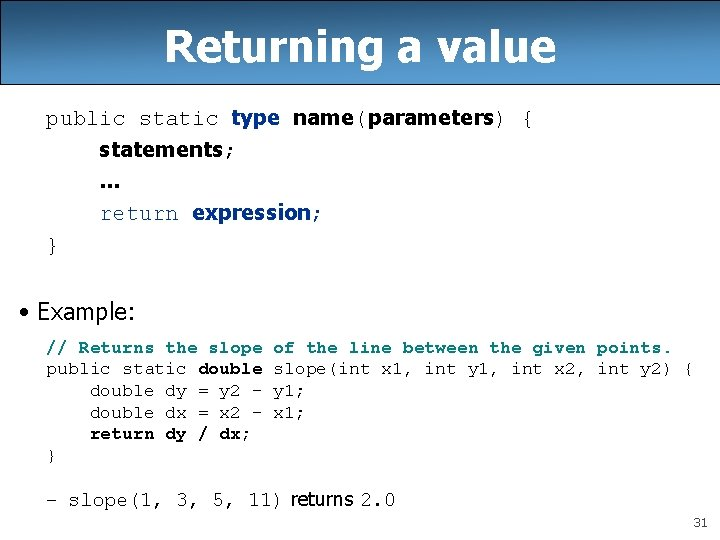Returning a value public static type name(parameters) { statements; . . . return expression; } • Example: // Returns the slope public static double dy = y 2 double dx = x 2 return dy / dx; } of the line between the given points. slope(int x 1, int y 1, int x 2, int y 2) { y 1; x 1; – slope(1, 3, 5, 11) returns 2. 0 31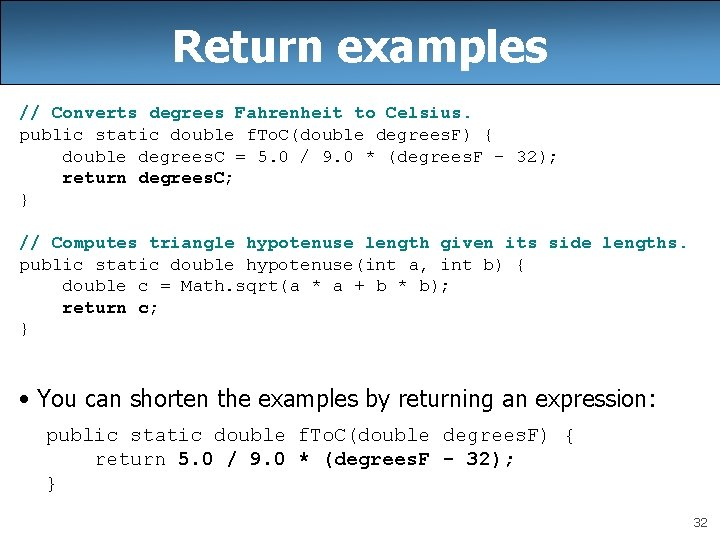Return examples // Converts degrees Fahrenheit to Celsius. public static double f. To. C(double degrees. F) { double degrees. C = 5. 0 / 9. 0 * (degrees. F - 32); return degrees. C; } // Computes triangle hypotenuse length given its side lengths. public static double hypotenuse(int a, int b) { double c = Math. sqrt(a * a + b * b); return c; } • You can shorten the examples by returning an expression: public static double f. To. C(double degrees. F) { return 5. 0 / 9. 0 * (degrees. F - 32); } 32Common error: Not storing • Many students incorrectly think that a return statement sends a variable's name back to the calling method. public static void main(String[] args) { slope(0, 0, 6, 3); System. out. println("The slope is " + result); // ERROR: } // result not defined public static double slope(int x 1, int x 2, int y 1, int y 2) { double dy = y 2 - y 1; double dx = x 2 - x 1; double result = dy / dx; return result; } 33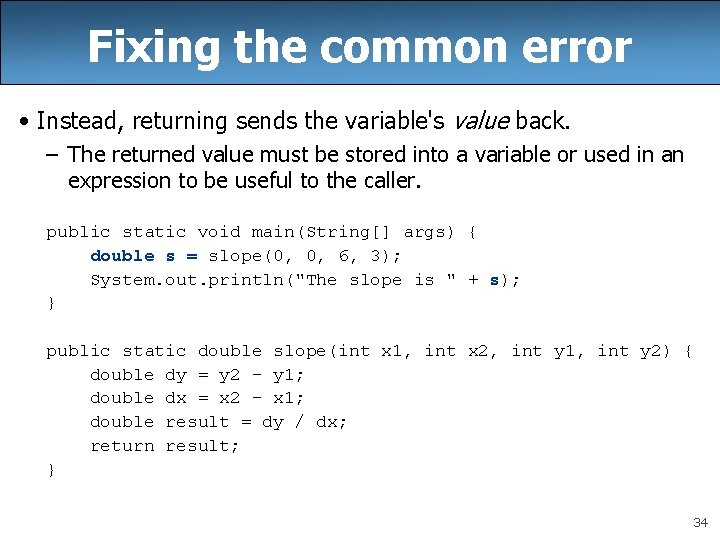Fixing the common error • Instead, returning sends the variable's value back. – The returned value must be stored into a variable or used in an expression to be useful to the caller. public static void main(String[] args) { double s = slope(0, 0, 6, 3); System. out. println("The slope is " + s); } public static double slope(int x 1, int x 2, int y 1, int y 2) { double dy = y 2 - y 1; double dx = x 2 - x 1; double result = dy / dx; return result; } 34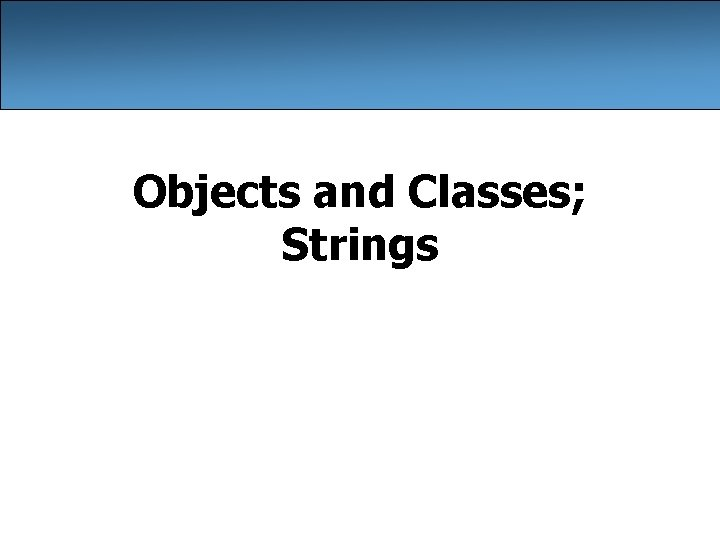Objects and Classes; Strings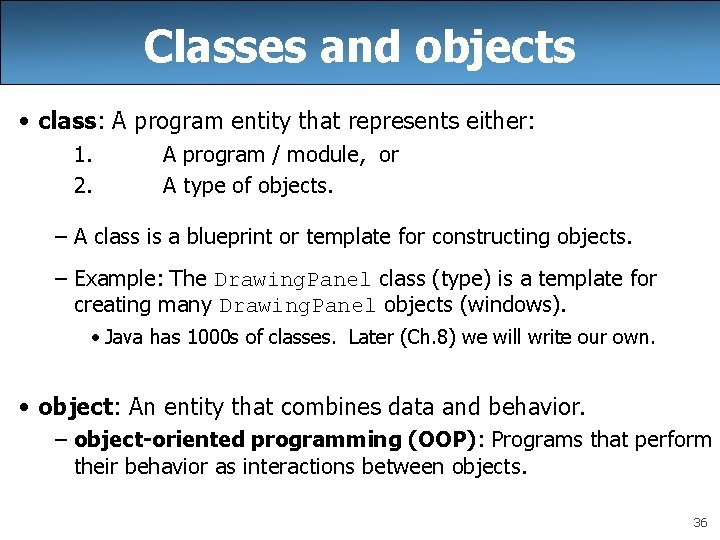Classes and objects • class: A program entity that represents either: 1. 2. A program / module, or A type of objects. – A class is a blueprint or template for constructing objects. – Example: The Drawing. Panel class (type) is a template for creating many Drawing. Panel objects (windows). • Java has 1000 s of classes. Later (Ch. 8) we will write our own. • object: An entity that combines data and behavior. – object-oriented programming (OOP): Programs that perform their behavior as interactions between objects. 36Objects • object: An entity that contains data and behavior. – data: variables inside the object – behavior: methods inside the object • You interact with the methods; the data is hidden in the object. • Constructing (creating) an object: Type object. Name = new Type(parameters); • Calling an object's method: object. Name. method. Name(parameters); 37Blueprint analogy i. Pod blueprint/factory state: current song volume battery life behavior: power on/off change station/song change volume choose random song creates i. Pod #1 i. Pod #2 i. Pod #3 state: song = "1, 000 Miles" volume = 17 battery life = 2. 5 hrs state: song = "Letting You" volume = 9 battery life = 3. 41 hrs state: song = "Discipline" volume = 24 battery life = 1. 8 hrs behavior: power on/off change station/song change volume choose random song 38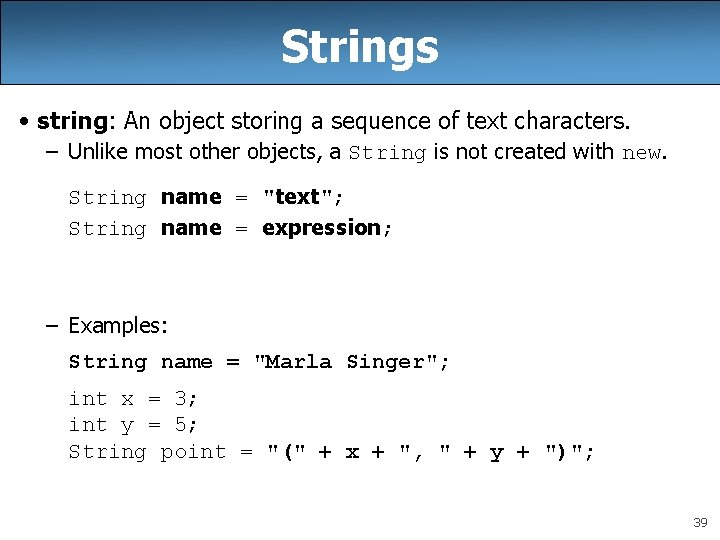Strings • string: An object storing a sequence of text characters. – Unlike most other objects, a String is not created with new. String name = "text"; String name = expression; – Examples: String name = "Marla Singer"; int x = 3; int y = 5; String point = "(" + x + ", " + y + ")"; 39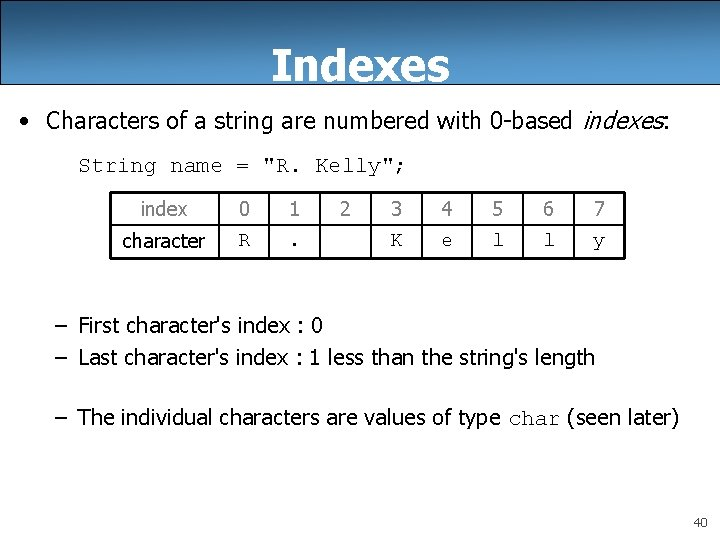Indexes • Characters of a string are numbered with 0 -based indexes: String name = "R. Kelly"; index 0 1 character R . 2 3 4 5 6 7 K e l l y – First character's index : 0 – Last character's index : 1 less than the string's length – The individual characters are values of type char (seen later) 40String methods Method name index. Of(str) Description index where the start of the given string appears in this string (-1 if not found) length() number of characters in this string substring(index 1, index 2) or substring(index 1) the characters in this string from index 1 (inclusive) to index 2 (exclusive); if index 2 is omitted, grabs till end of string to. Lower. Case() a new string with all lowercase letters to. Upper. Case() a new string with all uppercase letters • These methods are called using the dot notation: String gangsta = "Dr. Dre"; System. out. println(gangsta. length()); // 7 41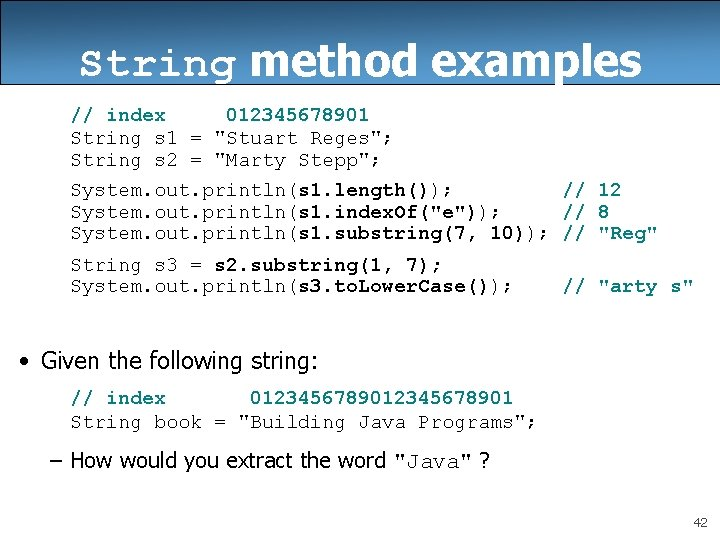String method examples // index 012345678901 String s 1 = "Stuart Reges"; String s 2 = "Marty Stepp"; System. out. println(s 1. length()); // 12 System. out. println(s 1. index. Of("e")); // 8 System. out. println(s 1. substring(7, 10)); // "Reg" String s 3 = s 2. substring(1, 7); System. out. println(s 3. to. Lower. Case()); // "arty s" • Given the following string: // index 012345678901 String book = "Building Java Programs"; – How would you extract the word "Java" ? 42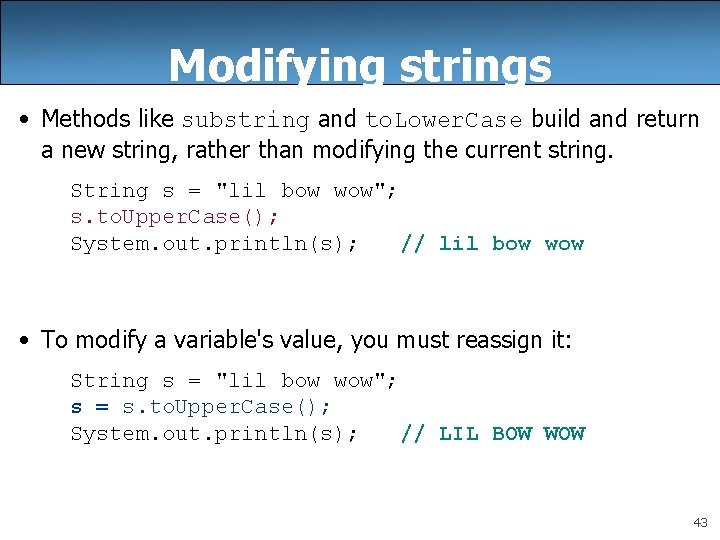Modifying strings • Methods like substring and to. Lower. Case build and return a new string, rather than modifying the current string. String s = "lil bow wow"; s. to. Upper. Case(); System. out. println(s); // lil bow wow • To modify a variable's value, you must reassign it: String s = "lil bow wow"; s = s. to. Upper. Case(); System. out. println(s); // LIL BOW WOW 43Interactive Programs with Scanner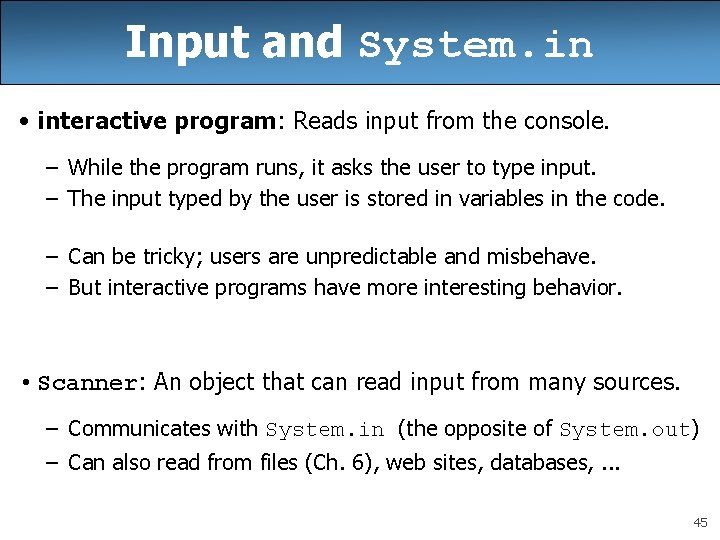Input and System. in • interactive program: Reads input from the console. – While the program runs, it asks the user to type input. – The input typed by the user is stored in variables in the code. – Can be tricky; users are unpredictable and misbehave. – But interactive programs have more interesting behavior. • Scanner: An object that can read input from many sources. – Communicates with System. in (the opposite of System. out) – Can also read from files (Ch. 6), web sites, databases, . . . 45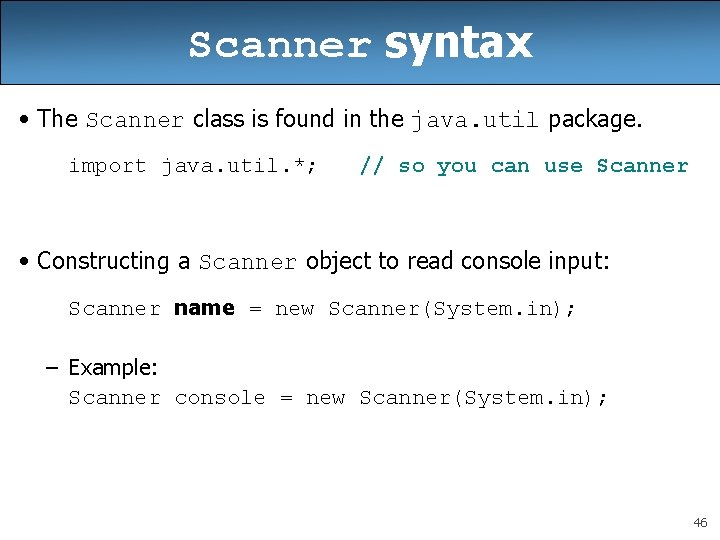Scanner syntax • The Scanner class is found in the java. util package. import java. util. *; // so you can use Scanner • Constructing a Scanner object to read console input: Scanner name = new Scanner(System. in); – Example: Scanner console = new Scanner(System. in); 46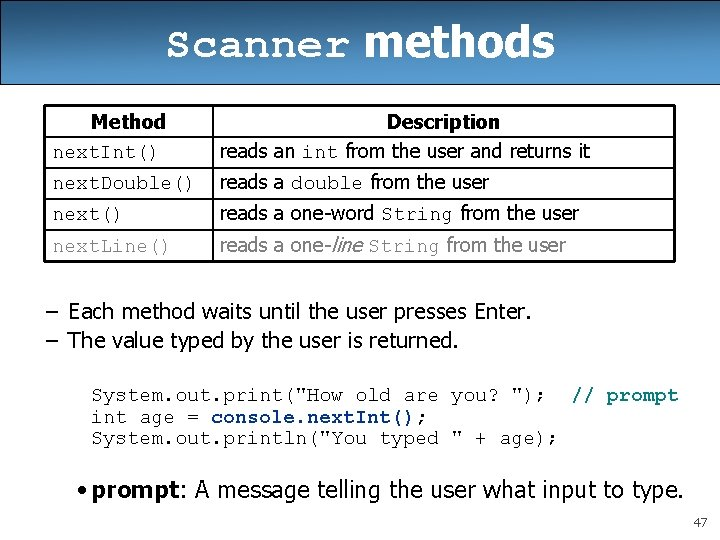Scanner methods Method next. Int() Description reads an int from the user and returns it next. Double() reads a double from the user next() reads a one-word String from the user next. Line() reads a one-line String from the user – Each method waits until the user presses Enter. – The value typed by the user is returned. System. out. print("How old are you? "); // prompt int age = console. next. Int(); System. out. println("You typed " + age); • prompt: A message telling the user what input to type. 47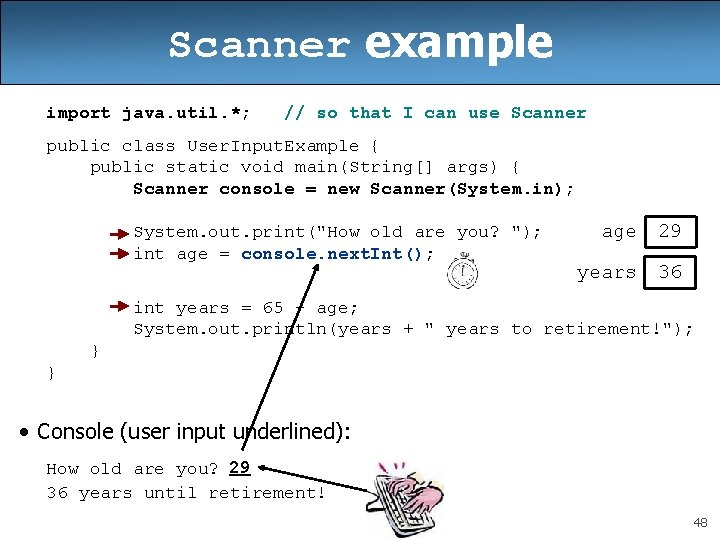Scanner example import java. util. *; // so that I can use Scanner public class User. Input. Example { public static void main(String[] args) { Scanner console = new Scanner(System. in); System. out. print("How old are you? "); int age = console. next. Int(); age 29 years 36 int years = 65 - age; System. out. println(years + " years to retirement!"); } } • Console (user input underlined): How old are you? 29 36 years until retirement! 48Scanner example 2 import java. util. *; // so that I can use Scanner public class Scanner. Multiply { public static void main(String[] args) { Scanner console = new Scanner(System. in); System. out. print("Please type two numbers: "); int num 1 = console. next. Int(); int num 2 = console. next. Int(); int product = num 1 * num 2; System. out. println("The product is " + product); } } • Output (user input underlined): Please type two numbers: 8 6 The product is 48 – The Scanner can read multiple values from one line. 49Input tokens • token: A unit of user input, as read by the Scanner. – Tokens are separated by whitespace (spaces, tabs, new lines). – How many tokens appear on the following line of input? 23 John Smith 42. 0 "Hello world" \$2. 50 " 19" • When a token is not the type you ask for, it crashes. System. out. print("What is your age? "); int age = console. next. Int(); Output: What is your age? Timmy java. util. Input. Mismatch. Exception at java. util. Scanner. next(Unknown Source) at java. util. Scanner. next. Int(Unknown Source). . . 50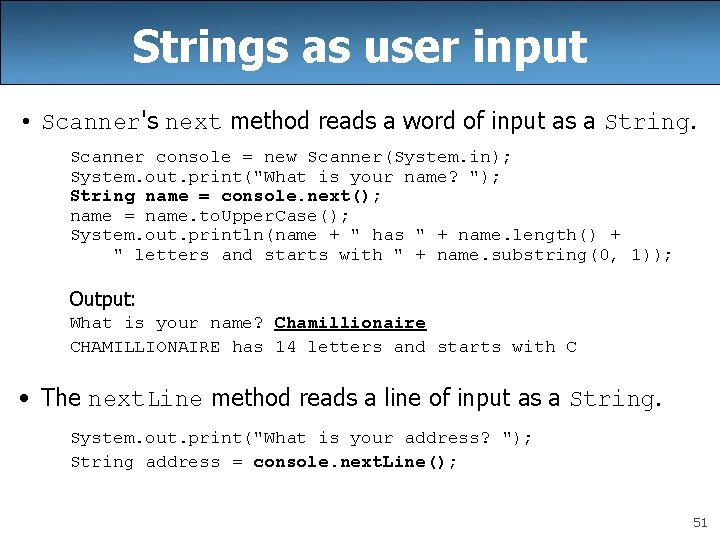Strings as user input • Scanner's next method reads a word of input as a String. Scanner console = new Scanner(System. in); System. out. print("What is your name? "); String name = console. next(); name = name. to. Upper. Case(); System. out. println(name + " has " + name. length() + " letters and starts with " + name. substring(0, 1)); Output: What is your name? Chamillionaire CHAMILLIONAIRE has 14 letters and starts with C • The next. Line method reads a line of input as a String. System. out. print("What is your address? "); String address = console. next. Line(); 51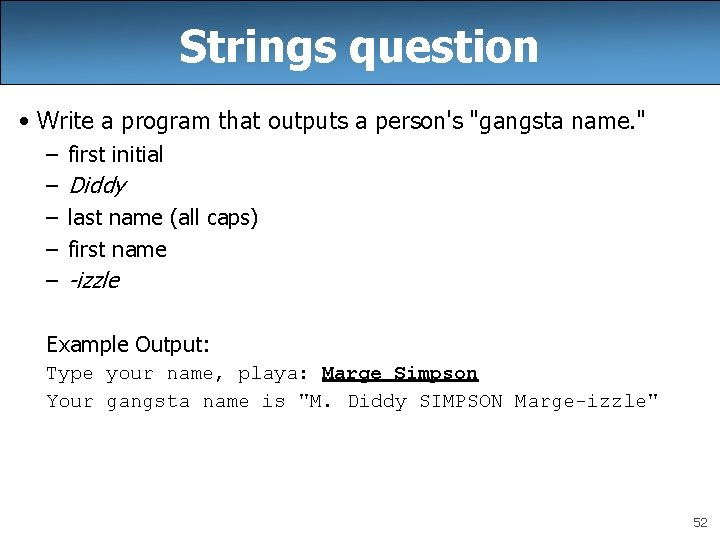Strings question • Write a program that outputs a person's "gangsta name. " – – – first initial Diddy last name (all caps) first name -izzle Example Output: Type your name, playa: Marge Simpson Your gangsta name is "M. Diddy SIMPSON Marge-izzle" 52Strings answer // This program prints your "gangsta" name. import java. util. *; public class Gangsta. Name { public static void main(String[] args) { Scanner console = new Scanner(System. in); System. out. print("Type your name, playa: "); String name = console. next. Line(); // split name into first/last name and initials String first = name. substring(0, name. index. Of(" ")); String last = name. substring(name. index. Of(" ") + 1); last = last. to. Upper. Case(); String f. Initial = first. substring(0, 1); System. out. println("Your gangsta name is "" + f. Initial + ". Diddy " + last + " " + first + "-izzle""); } } 53